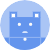search
Search
menu search toc more_vert
Guest 0reps
Thanks for the thanks!
close
account_circle
Profile
exit_to_app
Sign out
help Ask a question
search
keyboard_voice
close
Searching Tips
Search for a recipe:
"Creating a table in MySQL"
Search for an API documentation: "@append"
Search for code: "!dataframe"
Apply a tag filter: "#python"
Useful Shortcuts
/ to open search panel
Esc to close search panel
to navigate between search results
d to clear all current filters
Enter to expand content previewDoc SearchCode Search BetaSORRY NOTHING FOUND!
mic
Start speaking...Voice search is only supported in Safari and Chrome.
Shrink
Navigate to
A
A
brightness_medium
share
arrow_backShareTwitterFacebook
0
thumb_down
0
chat_bubble_outline
0
auto_stories new
settings

# Pandas | qcut method

Pandas
chevron_right
Documentation
chevron_right
General Functions
schedule Jul 1, 2022
Last updated
local_offer PandasPython
Tags

Pandas' `qcut(~)` method categorises numerical values into quantile bins (intervals) such that the number of items in each bin is equivalent.

# Parameters

1. `x`link | `array-like`

A 1D input array whose numerical values will be segmented into bins.

2. `q`link | `int` or `sequence<number>` or `IntervalIndex`

The number of quantiles. If `q=4`, then quartiles will be computed. You could also pass in an array of quartiles (e.g. `[0, 0.1, 0.5, 1]`].

3. `labels`link | `array` or `False` | `optional`

The desired labels of the bins. By default, `labels=None`.

4. `retbins`link | `boolean` | `optional`

Whether or not to return bins. By default, `retbins=False`.

5. `precision`link | `int` | `optional`

The number of decimal places to include up until for the bin labels. By default, `precision=3`.

6. `duplicates`link | `string` | `optional`

How to deal with duplicate bin edges:

Value

Description

`"raise"`

Throw an error if any duplicate bin edges are set.

`"drop"`

Remove the duplicate bin edge and just keep one.

By default, `duplicates="raise"`.

# Return Value

If `retbins=False`, then the return type depends on the value of the `labels` parameter:

• If `labels` is unspecified, then a `Series` or `Categorical` that encode the bins for each value is returned.

• If an array is supplied, then a `Series` or `Categorical` is returned.

• If a boolean `False` is supplied, then a NumPy array of integers is returned.

If `retbins=True`, then in addition to the above, the bins are returned as a NumPy array. If `x` is an `IntervalIndex`, then `x` is returned instead.

# Examples

Consider the following DataFrame about students and their grades:

``` raw_grades = [3,6,8,7,3,5]students = ["alex", "bob", "cathy", "doge", "eric", "fred"]df = pd.DataFrame({"name":students,"raw_grade":raw_grades})df    name  raw_grade0  alex     31  bob      62  cathy    83  doge     74  eric     35  fred     5 ```

## Basic usage

To categorise the raw grades into four bins (segments):

``` df["grade"] = pd.qcut(df["raw_grade"], q=4)df    name  raw_grade     grade0  alex     3      (2.999, 3.5]1  bob      6      (5.5, 6.75]2  cathy    8      (6.75, 8.0]3  doge     7      (6.75, 8.0]4  eric     3      (2.999, 3.5]5  fred     5      (3.5, 5.5] ```

The four quartiles here are as follows:

``` 1st: (2.999, 3.5]2nd: (3.5, 5.5]3rd: (5.5, 6.75]4th: (6.75, 8.0] ```

Note that `(2.995, 3.5]` just means that the `2.999 < raw_grade <= 3.5`.

## Specifying quartiles

To specify custom quartiles, we can pass in an `array` of quartiles instead of an `int`:

``` df["grade"] = pd.qcut(df["raw_grade"], q=[0, .4, .8, 1])df    name  raw_grade    grade0  alex     3      (2.999, 5.0]1  bob      6      (5.0, 7.0]2  cathy    8      (7.0, 8.0]3  doge     7      (5.0, 7.0]4  eric     3      (2.999, 5.0]5  fred     5      (2.999, 5.0] ```

## Specifying labels

We can give labels to our bins by setting the `labels` parameter:

``` df["grade"] = pd.qcut(df["raw_grade"], q=4, labels=["D","C","B","A"])df    name  raw_grade  grade0  alex     3         D1  bob      6         B2  cathy    8         A3  doge     7         A4  eric     3         D5  fred     5         C ```

This is an extremely practical feature of the `qcut(~)` method. Here, the length of the `labels` array must equal the specified number of quartiles.

## Specifying retbins

To get the computed bin edges as well, set `retbins=True`:

``` x = [3,6,8,7,4,5]res = pd.cut(x, bins=2, retbins=True)print("Categories: ", res)print("Bin egdes: ", res) Categories: [(2.999, 4.5], (4.5, 6.0], (6.75, 8.0], (6.75, 8.0], (2.999, 4.5], (4.5, 6.0]]Categories (4, interval[float64]): [(2.999, 4.5] < (4.5, 6.0] < (6.0, 6.75] < (6.75, 8.0]]Bin egdes: [ 3. 4.5 6. 6.75 8. ] ```

## Specifying precision

In order to control how many decimal places are displayed, set the `precision` parameter:

``` x = [3,6,8,7,4,5]bins = pd.qcut(x, q=4, precision=2)print(bins) [(2.99, 4.25], (5.5, 6.75], (6.75, 8.0], (6.75, 8.0], (2.99, 4.25], (4.25, 5.5]]Categories (4, interval[float64]): [(2.99, 4.25] < (4.25, 5.5] < (5.5, 6.75] < (6.75, 8.0]] ```

Here, `2.999` got truncated to `2.99` since we set a `precision` of `2`.

## Specifying duplicates

By default, the bin edges must be unique, otherwise an error will be thrown. For instance:

``` x = [3,6,8,7,3,5]pd.qcut(x, q=5) # duplicates="raise" ValueError: Bin edges must be unique: array([ 3., 3., 5., 6., 7., 8.]). ```

Here, we ended up with two bin edges of value 3, so that's why we get an error.

In order to drop (remove) redundant bin edges, set `duplicates="drop"`, like so:

``` x = [3,6,8,7,3,5]pd.qcut(x, q=5, duplicates="drop") [(2.999, 5.0], (5.0, 6.0], (7.0, 8.0], (6.0, 7.0], (2.999, 5.0], (2.999, 5.0]]Categories (4, interval[float64]): [(2.999, 5.0] < (5.0, 6.0] < (6.0, 7.0] < (7.0, 8.0]] ```
mail